# How to read a metric vernier caliper?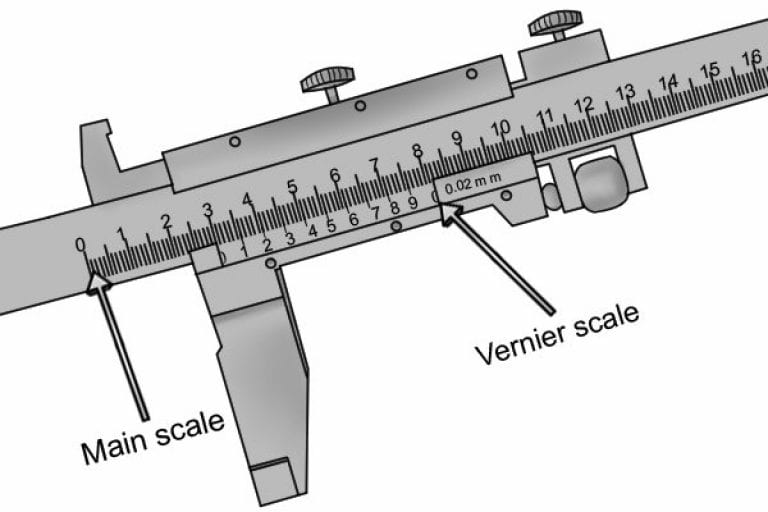If you are used to using a dial caliper, it can be a little tricky to get used to reading a vernier caliper. However, once you get the hang of it, it is a straightforward way of taking measurements quickly and accurately!

Although the vernier caliper can be used to measure a variety of different dimensions, the way in which it is read is always the same.

The reading on a vernier caliper is a combination of the value shown on the main scale and the value indicated on the sliding vernier scale.

## Guide to Reading a Metric Vernier Caliper

### Step 1 – Look at the main scale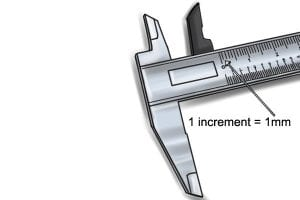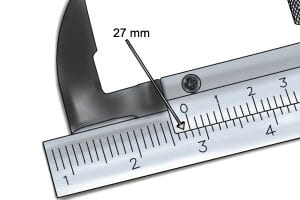When taking a measurement, you should first read the value on the main scale. On a metric vernier caliper, this will be given in millimetres (mm).

The smallest value that can read from the main scale is 1mm (indicated by a single increment).

The value on the main scale is the number immediately to the left of the 0 marker of the vernier scale.

In this instance, this value is 27mm.

As the zero is almost touching the 28mm mark, we can estimate that the distance between the jaws is closer to 28mm than 27mm.

When taking a measurement, you should first read the value on the main scale. On a metric vernier caliper, this will be given in millimetres (mm).

The smallest value that can read from the main scale is 1mm (indicated by a single increment).The value on the main scale is the number immediately to the left of the 0 marker of the vernier scale.

In this instance, this value is 27mm.

As the zero is almost touching the 28mm mark, we can estimate that the distance between the jaws is closer to 28mm than 27mm.### Step 2 – Look at the vernier scale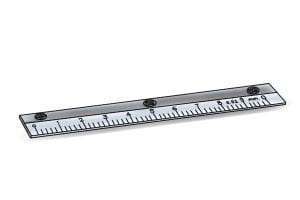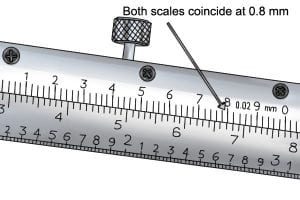Next, look at the vernier scale. The vernier scale of a metric vernier caliper has a measuring range of 1 mm. In the example we will be looking at, the vernier scale is graduated in 50 increments.

Each increment represents 0.02mm. However, some vernier scales are graduated in 20 increments, with each one representing 0.05mm.

When reading the vernier scale, identify the increment that lines up most accurately with an increment on the main scale. This value will make up the second part of your measurement.

In the example, the increments of both scales coincide at the 0.8mm on the vernier scale.

Next, look at the vernier scale. The vernier scale of a metric vernier caliper has a measuring range of 1 mm. In the example we will be looking at, the vernier scale is graduated in 50 increments.

Each increment represents 0.02mm. However, some vernier scales are graduated in 20 increments, with each one representing 0.05mm.When reading the vernier scale, identify the increment that lines up most accurately with an increment on the main scale. This value will make up the second part of your measurement.

In the example, the increments of both scales coincide at the 0.8mm on the vernier scale.### Step 3 – Add both values together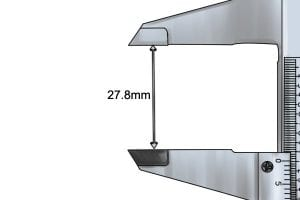To get your total reading, add both the value from the main scale and the value from the vernier scale together.

e.g. 27 + 0.8 = 27.8

So, the distance between the jaws of the vernier caliper is 27.8mm.

To get your total reading, add both the value from the main scale and the value from the vernier scale together.

e.g. 27 + 0.8 = 27.8

So, the distance between the jaws of the vernier caliper is 27.8mm.